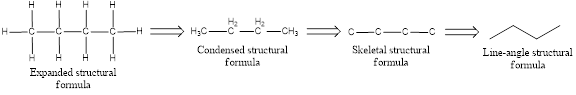Chapter 3, Problem 3.17EP### Organic And Biological Chemistry

7th Edition
STOKER + 1 other
ISBN: 9781305081079

#### Solutions

Chapter
Section### Organic And Biological Chemistry

7th Edition
STOKER + 1 other
ISBN: 9781305081079
Textbook Problem

# Write a condensed structural formula for each of the following unsaturated alcohols. a. 4-Penten-2-ol b. l-Pentyn-3-ol c. 3-Methyl-3-buten-2-ol d. 3-Buten-l-ol

(a)

Interpretation Introduction

Interpretation:

Condensed structural formula has to be written for the given unsaturated alcohol.

Concept Introduction:

The structural representation of organic compound can be done in 2D and 3D. In two-dimensional representation, there are four types of representation in which an organic compound can be drawn. They are,

• Expanded structural formula
• Condensed structural formula
• Skeletal structural formula
• Line-angle structural formula

Structural formula which shows all the atoms in a molecule along with all the bonds that is connecting the atoms present in the molecule is known as Expanded structural formula.

Structural formula in which grouping of atoms are done and in which the central atoms along with the other atoms are connected to them are treated as group is known as Condensed structural formula.

Structural formula that shows the bonding between carbon atoms alone in the molecule ignoring the hydrogen atoms being shown explicitly is known as Skeletal structural formula.

Structural formula where a line represent carbon‑carbon bond and the carbon atom is considered to be present in each point and the end of lines is known as Line-angle structural formula.Explanation

Given name of alcohol is 4-penten-2-ol.

From the name it is identified that the parent carbon chain is pentane and this possess a hydroxyl functional group on first second carbon atom with a double bond between fourth and fifth carbon atom. The structure can be drawn as,

(b)

Interpretation Introduction

Interpretation:

Condensed structural formula has to be written for the given unsaturated alcohol.

Concept Introduction:

The structural representation of organic compound can be done in 2D and 3D. In two-dimensional representation, there are four types of representation in which an organic compound can be drawn. They are,

• Expanded structural formula
• Condensed structural formula
• Skeletal structural formula
• Line-angle structural formula

Structural formula which shows all the atoms in a molecule along with all the bonds that is connecting the atoms present in the molecule is known as Expanded structural formula.

Structural formula in which grouping of atoms are done and in which the central atoms along with the other atoms are connected to them are treated as group is known as Condensed structural formula.

Structural formula that shows the bonding between carbon atoms alone in the molecule ignoring the hydrogen atoms being shown explicitly is known as Skeletal structural formula.

Structural formula where a line represent carbon‑carbon bond and the carbon atom is considered to be present in each point and the end of lines is known as Line-angle structural formula.(c)

Interpretation Introduction

Interpretation:

Condensed structural formula has to be written for the given unsaturated alcohol.

Concept Introduction:

The structural representation of organic compound can be done in 2D and 3D. In two-dimensional representation, there are four types of representation in which an organic compound can be drawn. They are,

• Expanded structural formula
• Condensed structural formula
• Skeletal structural formula
• Line-angle structural formula

Structural formula which shows all the atoms in a molecule along with all the bonds that is connecting the atoms present in the molecule is known as Expanded structural formula.

Structural formula in which grouping of atoms are done and in which the central atoms along with the other atoms are connected to them are treated as group is known as Condensed structural formula.

Structural formula that shows the bonding between carbon atoms alone in the molecule ignoring the hydrogen atoms being shown explicitly is known as Skeletal structural formula.

Structural formula where a line represent carbon‑carbon bond and the carbon atom is considered to be present in each point and the end of lines is known as Line-angle structural formula.(d)

Interpretation Introduction

Interpretation:

Condensed structural formula has to be written for the given unsaturated alcohol.

Concept Introduction:

The structural representation of organic compound can be done in 2D and 3D. In two-dimensional representation, there are four types of representation in which an organic compound can be drawn. They are,

• Expanded structural formula
• Condensed structural formula
• Skeletal structural formula
• Line-angle structural formula

Structural formula which shows all the atoms in a molecule along with all the bonds that is connecting the atoms present in the molecule is known as Expanded structural formula.

Structural formula in which grouping of atoms are done and in which the central atoms along with the other atoms are connected to them are treated as group is known as Condensed structural formula.

Structural formula that shows the bonding between carbon atoms alone in the molecule ignoring the hydrogen atoms being shown explicitly is known as Skeletal structural formula.

Structural formula where a line represent carbon‑carbon bond and the carbon atom is considered to be present in each point and the end of lines is known as Line-angle structural formula.### Still sussing out bartleby?

Check out a sample textbook solution.

See a sample solution

#### The Solution to Your Study Problems

Bartleby provides explanations to thousands of textbook problems written by our experts, many with advanced degrees!

Get Started